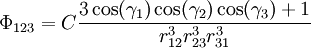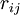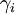# Axilrod-Teller interactionThis article is a 'stub' page, it has no, or next to no, content. It is here at the moment to help form part of the structure of SklogWiki. If you add sufficient material to this article then please remove the {{Stub-general}} template from this page.$\Phi_{123}= C\frac{3 \cos(\gamma_1)\cos(\gamma_2) \cos(\gamma_3) +1}{r_{12}^3 r_{23}^3 r_{31}^3}$
where$r_{ij}$ are the lengths of the sides and$\gamma_i$ are the angles of the triangle formed by the three atoms.